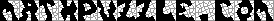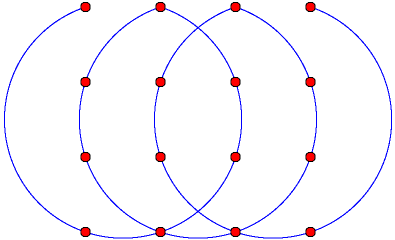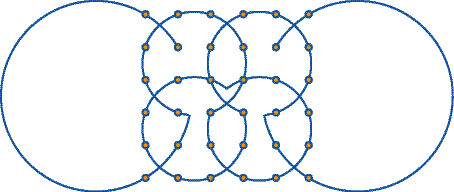This header plots the critical line of the Riemann Zeta Function.  A complete understanding wins a \$1,000,000 prize.
 . . .Main Links Orders Post Next Page Next + 10

Connect the dots - Arcs

Erich Friedman made a page about Circles through Points. David Eppstein (Geometry Junkyard) suggested combining these problems. What is the fewest number of continuous arcs needed to pass through a grid of points? David shows that three arcs are enough for the 4x4 case. What's the answer for the 5x5?Koshi Arai was the first solver, ever. Koshi was followed closely by Joseph DeVincentis and Roger Phillips, who sent different solutions. All three solutions require at least one tricky circle. Ignacio Ruiz de Conejo and Adrian Fisher also found solutions. Adrian found a solution with 4 arcs through a side-5 triangular grid. Koshi, Joe, and Roger solved a 6x6 array of dots with 6 arcs. Koshi's solution is below. Joe and Roger found a solution that ends at the starting point. Can you match them? Higher order grids are still unsolved.Koshi Arai was the first to solve the 5x5 problem with 5 arcsJoseph DeVincentis modified a solution by Koshi Arai to be continuous.Roger Phillips shows that 9 arcs can solve the 7x7 grid. Can this be solved with 8 arcs?Connect the dots - Lines

Brain games such as Connect the dots are definitely one of the most famous and favorite games available in the net. Lately Brain games also entered the world of Online Casino brain games with developments of new games called "Guess the Game" or similar. An interesting alternative and fun gaming experience.

Michael Cysouw[MC], Roger Phillips{RP], Toshi Kato[TK], and Joseph Devincentis[JD] have all made nice discoveries.  The 6x6 puzzle was published in a Japanese book by Gisaku Nakamura.  Harry Nelson has informed me that most of these problems were discussed by Solomon Golomb in Journal of Rec Math, Vol 1 No. 3 pps154-155.

Based on all these results, I'll say that a closed path of size 2*(n-1) exists for all grids of size n*n for n>2.  As Roger Phillips points out, this is always one less line than the trivial path.  Conjecture: No path with two lines less than the trivial path  is possible, closed or otherwise.  Counterexamples, anyone?

These are all 'connect-the-dot' problems.  In all examples, you must use a continuous series of lines, and may not lift your pencil off the paper as you connect the centers of a grid of dots.  (Without centers, Spital offers this)
1.  Using four lines, connect the dots of a 3x3 grid (answer).
2.  Using five lines, connect the dots of a 3x4 grid, ending at the place you started, and not visiting any dot twice (answer).
3.  Using six lines, connect the dots of a 4x4 grid, ending where you started, and not visiting any dot twice (answer).
4.  Using seven lines, connect the dots of a 4x5 grid, ending where you started, and not visiting any dot twice (MC answer).
5.  Using eight lines, connect the dots of a 5x5 grid (MC answer), ending where you started (TK answer).
6.  Using nine lines, connect the dots of a 5x6 grid, ending where you started, and not visiting any dot twice (MC answer).
7.  Using ten lines, connect the dots of a 6x6 grid, ending where your started (MC answer1) , and not visiting any dot twice. (JD/TK answer2, RP answer3)
8.  Using twelve lines, connect the dots of a 7x7 grid (MC answer1, answer2), ending where you started (TK answer).
9.  Using fourteen lines, connect the dots of an 8x8 grid (answer), ending where you started and not using any dot twice (TK answer).
10.  Using sixteen lines, connect the dots of a 9x9 grid, ending where you started (TK answer).
10,  Using eightteen lines, connect the dots of a 10x10 grid, ending where you started, and not visiting any dot twice (JD & TK answer1, RP answer2).

Many of these answers amaze me.  You should try to solve it yourself before looking at the solution.  An ideal solution uses the minimal number of lines, never goes through the same dot twice, and ends at the place it started (re-entrant).

Michael Cysouw conjectures:
Closed paths without visiting any dot twice can be made for:
- 2a x 2a grids, using a closed graph of 2(2a-1) lines
- a x (a+1) grids, using a closed graph of 2a-1 lines
- all other grids are trivial (meaning: there are no interesting improvements possible to an easily to be discovered closed path)

Toshi Kato conjectures:
On (2N+1)x(2N+1) grid, N>=2, Using 4N continuous lines, and not lifting your pencil from the paper, can go through all the dots of a (2N+1)x(2N+1) grid, ending at the same place started. But must visit at least one dot twice in the route.

On (2N)x(2N) grid, N>=2, Using 4N-2 continuous lines, and not lifting your pencil from the paper, can go through all the dots of a (2N)x(2N) grid, ending at the same place started. And can visit each dots just once.

Here is an algorithm by Roger Phillips:
Path for a square of n x n dots, where n = 2m, m >= 3: path is reentrant, using 2(n - 1) segments.
Let the bottom-left corner of the square be the origin (0, 0), so the top-right corner is (2m - 1, 2m - 1).
1. From the point (m - 1, 0) draw a line upwards with a gradient of  m - 1, to the top of the grid.
2. From the points (m - 1, 1), (m - 1, 2), ... (m - 1, m - 2), draw parallel (m - 1)-gradient lines to the top of the grid.
3. Draw lines right through the leftmost and rightmost m - 2 columns, ie join (0, 0) to (0, 2m - 1), (1, 0) to (1, 2m - 1), ... (m - 3, 0) to (m - 3, 2m - 1).
4. From (m - 2, 0), draw a line up to (m - 2, 2m - 3) (ie not through the top two dots), and then draw a line with gradient 1 up to the top of the grid,  ie joining (m - 2, 2m - 3) to (m, 2m - 1).
5. For each of the m - 1 lines drawn so far, draw its reflection in the grid's vertical axis of symmetry.

We now have 2(n - 1) segments, with each dot visited once, but we need to join up the segments to make a path. Consider the area above the left-hand half of the grid: we have m - 2 vertical lines emerging from the top of the grid, m - 1 parallel diagonal lines (gradient = -(m - 1)) coming out to meet them, plus one diagonal (gradient = -1) approaching the parallel lines from the right. There are many ways in which we can match up these lines, to form variations on the path. Arbitrarily, I'll choose the following method:

6. Extend the rightmost -(m - 1)-gradient diagonal and the -1-gradient diagonal until they meet.
7. Extend the leftmost of the remaining m - 2 diagonals of gradient -(m - 1) upward to meet the leftmost of the verticals. Extend the next diagonal to meet the next vertical, and so on for the rest of the diagonals.

Now consider the area above the right-hand half of the grid. It's the mirror image of what we had on the left-hand side, and again there are many possible variations on how to link up the loose ends, so I arbitrarily choose the mirror image of the method we used on the left.

Now consider the area below the left-hand half of the grid. It's tidier than the top was: we just have m - 1 verticals emerging from the bottom of the grid, and m - 1 diagonals approaching them from the right. Again, we could link them up in many different ways, so I arbitrarily choose a similar method to before:

8. Extend the leftmost of the (m - 1)-gradient diagonals down to meet the leftmost of the verticals. Extend the next diagonal down to meet the next vertical, and so on for the rest of the diagonals.

This leaves us with verticals descending from the bottom of the rightmost m - 1 columns of the grid, and m - 1 diagonals approaching them from the left. These are now the endpoints of m - 1 disjoint paths. If we number the endpoints of the diagonals from the left 1, 2, ... m - 1, then the other ends of the paths appear at the bottom of the verticals, numbered, from left to right, in the order m - 1, 2, 3, ... m - 2, 1, ie the same order except that the first and last are swapped. If we matched them up by the same method as before (ie 1 to m - 1, 2 to 2, 3 to 3, ... m - 2 to m - 2, m - 1 to 1), we'd clearly end up with separate closed paths for 2, 3, ... m - 2. There are still many ways we could join them up to make a single path, and I arbitrarily choose the following method (simply cycling the numbers of the endpoints by one place):

9. Join the (leftmost) diagonal 1 to the (second-leftmost) vertical 2, diagonal 2 to vertical 3, and so on to diagonal m - 3 and vertical m - 2.
10. Join diagonal m - 2 (the second-rightmost) to vertical m - 1 (the leftmost).
11. Join (the remaining) diagonal m - 1 to (the remaining) vertical 1.

Joseph DeVincentis offers this method:
The method I used for the 6x6 is extensible to some larger sizes.
Take an n x n-2 grid and draw the (ordinary 45-degree) diagonals
from each corner across it, and every second diagonal from these
off toward the small ones in the corners.  Extend every diagonal
a half unit beyond the last dot on it, so that all the ends but
the four on the corners of the grid meet.  Extend those last
four ends an additional half unit and connect them, passing
through those remaining 2 rows of points needed to make an n x n
grid.

For odd sizes, the diagonals in different directions cross at
the dots instead of between them.  For multiple-of-four sizes,
you end up making 2 separate loops instead of a single long
one in the last step.  For n=4k+2 sizes, this seems to work,
giving a re-entrant path which goes through each dot only once,
in 2n-2 lines.  Try it for n=10 and 14!